# php基础教程 第五步 逻辑控制

## if 语句

if语句的语法如下：

<?php
if(条件)
{
条件判断正确在此处输入需要执行的代码;
}
?>


<?php
if(2>1)
{
echo '2比1大';
}
?>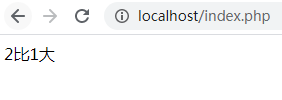<?php
if(1>2)
{
echo '2比1大';
}
?>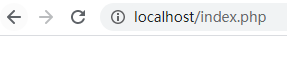## if else 语句

else语句用来说明当if条件判断不成立后，执行的代码语句。

<?php
if(1>2)
{
echo '2比1大';
}else{
echo '2比1大 判断错误';
}
?>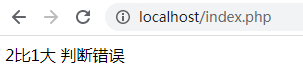<?php
if(1>2)
{
echo '判断正确 ';
echo '2比1大';
}else{
echo '判断错误 ';
echo '2比1大';
}
?>


<?php
$a=3;$b=5;
if($a>$b)
{
echo '判断正确 ';
echo $a.'比'.$b.'大';
}else{
echo '判断错误 ';
echo $a.'比'.$b.'小';
}
?>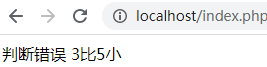## 或运算||

<?php
$a=3;$b=5;
if($a>$b||$a==3) { echo '判断正确 '; echo$a.'比'.$b.'大 或者变量a 等于3'; }else{ echo '判断错误 '; echo$a.'比'.$b.'小'; } ?>  以上代码中，在if条件内进行了更改：$a>$b||$a==3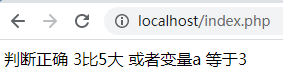<?php
$a=4;$b=5;
if($a>$b||$a==3||$a==4)
{
echo '判断正确 ';
echo $a.'比'.$b.'大 或者变量a 等于3、4';
}else{
echo '判断错误 ';
echo $a.'比'.$b.'小';
}
?>


$b=5; if($a>$b&&$a==11)
{
echo '判断正确 ';
echo $a.'比'.$b.'大 并且等于 11';
}else{
echo '判断错误 ';
echo $a.'比'.$b.'小';
}
?>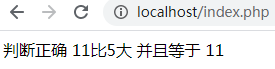## if…elseif…else语句

<?php
$a='天气大太阳'; if($a=='天气下雨')
{
echo '我在家看电视';
}elseif($a=='天气大太阳'){ echo '我晒衣服'; }elseif($a=='天气刮风'){
echo '我出吹风';
}
?>


elseif 则是使用在多个条件判断时，当满足不了其一，那么就接下去进行判断，直到找到符合条件成立的模块代码，随后执行。如果都没有找到条件成立的代码，那么这个if条件语句将什么都不执行。<?php
$a='天气下冰雹'; if($a=='天气下雨')
{
echo '我在家看电视';
}elseif($a=='天气大太阳'){ echo '我晒衣服'; }elseif($a=='天气刮风'){
echo '我们出吹风';
}else{
echo '我什么都不做';
}
?>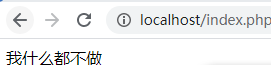## if语句嵌套

if语句是可以嵌套的，如下代码：

<?php
$a='天气下冰雹';$b='我妈不给我出门';
if($a=='天气下冰雹') { echo '天气下冰雹了'; if($b=='我妈给我出门'){
echo '出门玩';
}else{
echo '我妈不给我出门';
}
}
?>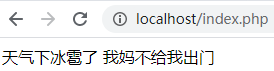php 文章持续更新中，欢迎关注、点赞、收藏、转发。

06-031万+
08-206107-131150
11-2135
07-141229
12-051196
05-3016
08-1150
08-13300
04-1049